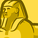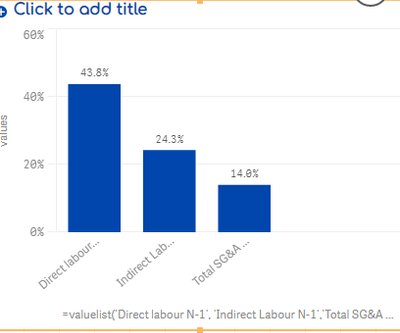# App Development

Announcements
Now Live: Qlik Sense SaaS Simplified Authoring – Analytics Creation for Everyone: READ DETAILS
cancel
Showing results for
Did you mean:Creator III

## Qliksense Pie chart showing addtitional values

Hello everyone,

I came accross a very strange behaviour below:-

I have below dimensions and measures :-

Dimension:- =valuelist('Direct labour N-1', 'Indirect Labour N-1','Total SG&A + R&D N-1')

Measure:-

if(valuelist('Direct labour N-1', 'Indirect Labour N-1','Total SG&A + R&D N-1')='Direct labour N-1',

num(sum({<Indicateur.Code = {'PO.1.161b'},[Site.isYFPO]={'0'},[Site.Participe]={'49|50'},Date.Year = , Date.MonthName = , Date.Quarter =, Date.Month =, Date.MonthEnd =, MonthYear=,Date=,NewTestvb1=,
Date= {"\$(=MonthStart(Max(Date)))"} >} [Indicateur.Value])
/

sum({<Indicateur.Code = {'PO.1.137b'},[Site.isYFPO]={'0'},[Site.Participe]={'49|50'},Date.Year = , Date.MonthName = , Date.Quarter =, Date.Month =, Date.MonthEnd =, MonthYear=,Date=,NewTestvb1=,
Date= {"\$(=MonthStart(Max(Date)))"}>} [Indicateur.Value])
,'###.#%'),

if(valuelist('Direct labour N-1', 'Indirect Labour N-1','Total SG&A + R&D N-1')='Indirect Labour N-1',
num(sum({<Indicateur.Code = {'PO.1.162b'},[Site.isYFPO]={'0'},[Site.Participe]={'49|50'},Date.Year = , Date.MonthName = , Date.Quarter =, Date.Month =, Date.MonthEnd =, MonthYear=,Date=,NewTestvb1=,
Date= {"\$(=MonthStart(Max(Date)))"} >} [Indicateur.Value])
/

sum({<Indicateur.Code = {'PO.1.137b'},[Site.isYFPO]={'0'},[Site.Participe]={'49|50'},Date.Year = , Date.MonthName = , Date.Quarter =, Date.Month =, Date.MonthEnd =, MonthYear=,Date=,NewTestvb1=,
Date= {"\$(=MonthStart(Max(Date)))"} >} [Indicateur.Value])
,'###.#%'),

if(valuelist('Direct labour N-1', 'Indirect Labour N-1','Total SG&A + R&D N-1')='Total SG&A + R&D N-1',

num(sum({<Indicateur.Code = {'PO.1.207b'},[Site.isYFPO]={'0'},[Site.Participe]={'49|50'},Date.Year = , Date.MonthName = , Date.Quarter =, Date.Month =, Date.MonthEnd =, MonthYear=,Date=,NewTestvb1=,
Date= {"\$(=MonthStart(Max(Date)))"} >} [Indicateur.Value])
/
sum({<Indicateur.Code = {'PO.1.137b'},[Site.isYFPO]={'0'},[Site.Participe]={'49|50'},Date.Year = , Date.MonthName = , Date.Quarter =, Date.Month =, Date.MonthEnd =, MonthYear=,Date=,NewTestvb1=,
Date= {"\$(=MonthStart(Max(Date)))"} >} [Indicateur.Value])
,'###.#%')

)

)
)

When i plot the above in bar chart , i get correct number s:-But when i created a pie chart, it is showing some additional values like below:-In the above  chart, the number corresponding to label 'Values-14.0%' is correct. However, there is an additional label named 'share-17.0%' which i have not calculated but somehow it is appearing out of nowhere.

I am unable to understand why exactly it is working fine in bar chart and causing problem in pie chart.

1 Solution

Accepted SolutionsCreator III
Author

Hello ,

I solved it myself by following below steps:-

1) Go to 'Apperance' - -> 'Presentation tab and do belowPost doing this, it will start showing you the value of your expression.

However, when you will hover on the chart, it will still you the value of Share%. In order to remove that, we make use of Qliksesne tool tip feature. I followed the below steps:

1)Go to tool tip, check the checkbox 'hide basic rows' and enter details of your dimensions and measuresEnd Result:- It is showing actual values of the expression and no value for share is displayed2 RepliesEmployee

I think that for a pie chart you should have numbers not %s for a measureCreator III
Author

Hello ,

I solved it myself by following below steps:-

1) Go to 'Apperance' - -> 'Presentation tab and do belowPost doing this, it will start showing you the value of your expression.

However, when you will hover on the chart, it will still you the value of Share%. In order to remove that, we make use of Qliksesne tool tip feature. I followed the below steps:

1)Go to tool tip, check the checkbox 'hide basic rows' and enter details of your dimensions and measuresEnd Result:- It is showing actual values of the expression and no value for share is displayed